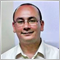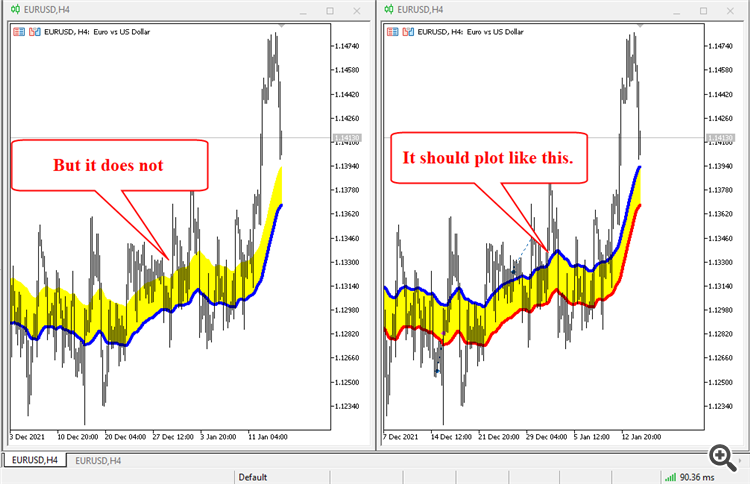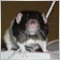DRAW FILLING Problem49127

Hi,

I want to build a moving average channel that fills the distance between its bands but for some reason, It is not plotted correctly on my MT5.

Here is a screenshot of what I want to do and what I get from my code..

Here is the code that I want to fix.

Note. I don't want to use the Program Properties (#property) ... I want to use the PlotIndexSetInteger() function.
.

#property version   "1.0"
#property indicator_chart_window
#property indicator_buffers 4
#property indicator_plots   3

//---BUFFERS
double Cloud1[], Cloud2[], HI[], LO[];

//--- Plot Handles

//=============================================================================================================
// ONINIT FUNCTION
//=============================================================================================================
int OnInit()
{
//+------------------------------------------------------------------+
//| Buffers Mapping                                                  |
//+------------------------------------------------------------------+
SetIndexBuffer(0, Cloud1, INDICATOR_DATA);
SetIndexBuffer(1, Cloud2, INDICATOR_DATA);
SetIndexBuffer(2, HI,     INDICATOR_DATA);
SetIndexBuffer(3, LO,     INDICATOR_DATA);

//--- indicator handles
HiHandle = iMA(NULL, PERIOD_CURRENT, 50, 0, MODE_EMA, PRICE_HIGH);
LoHandle = iMA(NULL, PERIOD_CURRENT, 50, 0, MODE_EMA, PRICE_LOW);

HiLineHandle = iMA(NULL, PERIOD_CURRENT, 50, 0, MODE_EMA, PRICE_HIGH);
LoLineHnadle = iMA(NULL, PERIOD_CURRENT, 50, 0, MODE_EMA, PRICE_LOW);

//+------------------------------------------------------------------+
//| Draw Begin & Digits                                              |
//+------------------------------------------------------------------+
for(int i = 1; i <= 4; i++) { PlotIndexSetInteger(i, PLOT_DRAW_BEGIN, 50); }

//--- indicator digits
IndicatorSetInteger(INDICATOR_DIGITS, _Digits);

//+------------------------------------------------------------------+
//| Short Name and Labels                                            |
//+------------------------------------------------------------------+
//---Index Short Name
string ShortName = "MA Channel ["+IntegerToString(50)+"]";
IndicatorSetString(INDICATOR_SHORTNAME, ShortName);

//---Index Data Window Label
PlotIndexSetString(0, PLOT_LABEL, "Cloud High");
PlotIndexSetString(1, PLOT_LABEL, "Cloud Low");
PlotIndexSetString(2, PLOT_LABEL, "MA High");
PlotIndexSetString(3, PLOT_LABEL, "MA Low");

//+------------------------------------------------------------------+
//| PLOT TYPE                                                        |
//+------------------------------------------------------------------+
PlotIndexSetInteger(0, PLOT_DRAW_TYPE, DRAW_FILLING);
PlotIndexSetInteger(1, PLOT_DRAW_TYPE, DRAW_NONE);
PlotIndexSetInteger(2, PLOT_DRAW_TYPE, DRAW_LINE);
PlotIndexSetInteger(3, PLOT_DRAW_TYPE, DRAW_LINE);

//+------------------------------------------------------------------+
//| PLOT COLOR                                                       |
//+------------------------------------------------------------------+
PlotIndexSetInteger(0,PLOT_LINE_COLOR, clrYellow);
PlotIndexSetInteger(1,PLOT_LINE_COLOR, clrYellow);
PlotIndexSetInteger(2,PLOT_LINE_COLOR, clrBlue);
PlotIndexSetInteger(3,PLOT_LINE_COLOR, clrRed);

//+------------------------------------------------------------------+
//| PLOT WIDTH                                                       |
//+------------------------------------------------------------------+
PlotIndexSetInteger(0, PLOT_LINE_WIDTH,1);
PlotIndexSetInteger(1, PLOT_LINE_WIDTH,1);
PlotIndexSetInteger(2, PLOT_LINE_WIDTH,4);
PlotIndexSetInteger(3, PLOT_LINE_WIDTH,4);

//+------------------------------------------------------------------+
//| PLOT LINE STYLE                                                  |
//+------------------------------------------------------------------+
PlotIndexSetInteger(0, PLOT_LINE_STYLE, STYLE_SOLID);
PlotIndexSetInteger(1, PLOT_LINE_STYLE, STYLE_SOLID);
PlotIndexSetInteger(2, PLOT_LINE_STYLE, STYLE_SOLID);
PlotIndexSetInteger(3, PLOT_LINE_STYLE, STYLE_SOLID);

//--- Set chart on foreground
ChartSetInteger(0,CHART_FOREGROUND, true);

//---
return(INIT_SUCCEEDED);
}

//=============================================================================================================
// ONCALCULATE FUNCTION
//=============================================================================================================
int OnCalculate(const int rates_total,
const int prev_calculated,
const datetime &time[],
const double &open[],
const double &high[],
const double &low[],
const double &close[],
const long &tick_volume[],
const long &volume[],
{
//---
if(IsStopped())                                {return(0);}
if(rates_total < 50)                           {return(0);}
if(BarsCalculated(HiHandle)     < rates_total) {return(0);}
if(BarsCalculated(LoHandle)     < rates_total) {return(0);}
if(BarsCalculated(HiLineHandle) < rates_total) {return(0);}

int CopyBars = 0;

if(prev_calculated < 0 || prev_calculated > rates_total)
{
CopyBars = rates_total;
} else {
CopyBars = rates_total - prev_calculated;
if(prev_calculated > 0) {CopyBars++;}
}

if(IsStopped())                                           {return(0);}
if(CopyBuffer(HiHandle,     0, 0, CopyBars, Cloud1) <= 0) {return(0);}
if(CopyBuffer(LoHandle,     0, 0, CopyBars, Cloud2) <= 0) {return(0);}
if(CopyBuffer(HiLineHandle, 0, 0, CopyBars, HI)     <= 0) {return(0);}
if(CopyBuffer(LoLineHnadle, 0, 0, CopyBars, LO)     <= 0) {return(0);}

//--- return value of prev_calculated for next call
return(rates_total);
}
//+------------------------------------------------------------------+

I attach the mq5 file.
Files:153

//+------------------------------------------------------------------+
//| PLOT TYPE                                                        |
//+------------------------------------------------------------------+
PlotIndexSetInteger(0, PLOT_DRAW_TYPE, DRAW_FILLING);
PlotIndexSetInteger(1, PLOT_DRAW_TYPE, DRAW_LINE);
PlotIndexSetInteger(2, PLOT_DRAW_TYPE, DRAW_LINE);

//+------------------------------------------------------------------+
//| PLOT COLOR                                                       |
//+------------------------------------------------------------------+
PlotIndexSetInteger(0,PLOT_LINE_COLOR, 0, clrYellow);
PlotIndexSetInteger(0,PLOT_LINE_COLOR, 1, clrYellow);
PlotIndexSetInteger(1,PLOT_LINE_COLOR, clrBlue);
PlotIndexSetInteger(2,PLOT_LINE_COLOR, clrRed);

• DRAW_FILLING takes two buffers, and then the next plot takes the next available after those — you don't have to declare DRAW_NONE
• DRAW_FILLING can take two different colors (changing depending on which value is greater) if needed49127

Haruto Rat #:

• DRAW_FILLING takes two buffers, and then the next plot takes the next available after those — you don't have to declare DRAW_NONE
• DRAW_FILLING can take two different colors (changing depending on which value is greater) if needed
Thanks for the explanation 🙏

I corrected the code:
#property version   "1.1"
#property indicator_chart_window
#property indicator_buffers 4
#property indicator_plots   3

//---BUFFERS
double Cloud1[], Cloud2[], HI[], LO[];

//--- Plot Handles

//=============================================================================================================
// ONINIT FUNCTION
//=============================================================================================================
int OnInit()
{
//+------------------------------------------------------------------+
//| Buffers Mapping                                                  |
//+------------------------------------------------------------------+
SetIndexBuffer(0, Cloud1, INDICATOR_DATA);
SetIndexBuffer(1, Cloud2, INDICATOR_DATA);
SetIndexBuffer(2, HI,     INDICATOR_DATA);
SetIndexBuffer(3, LO,     INDICATOR_DATA);

//--- indicator handles
HiHandle = iMA(NULL, PERIOD_CURRENT, 50, 0, MODE_EMA, PRICE_HIGH);
LoHandle = iMA(NULL, PERIOD_CURRENT, 50, 0, MODE_EMA, PRICE_LOW);

HiLineHandle = iMA(NULL, PERIOD_CURRENT, 50, 0, MODE_EMA, PRICE_HIGH);
LoLineHnadle = iMA(NULL, PERIOD_CURRENT, 50, 0, MODE_EMA, PRICE_LOW);

//+------------------------------------------------------------------+
//| Draw Begin & Digits                                              |
//+------------------------------------------------------------------+
for(int i = 0; i <= 2; i++) { PlotIndexSetInteger(i, PLOT_DRAW_BEGIN, 50); }

//--- indicator digits
IndicatorSetInteger(INDICATOR_DIGITS, _Digits);

//+------------------------------------------------------------------+
//| Short Name and Labels                                            |
//+------------------------------------------------------------------+
//---Index Short Name
string ShortName = "MA Channel ["+IntegerToString(50)+"]";
IndicatorSetString(INDICATOR_SHORTNAME, ShortName);

//---Index Data Window Label
PlotIndexSetString(0, PLOT_LABEL, "MA Cloud");
PlotIndexSetString(1, PLOT_LABEL, "MA High");
PlotIndexSetString(2, PLOT_LABEL, "MA Low");

//+------------------------------------------------------------------+
//| PLOT TYPE                                                        |
//+------------------------------------------------------------------+
PlotIndexSetInteger(0, PLOT_DRAW_TYPE, DRAW_FILLING);
PlotIndexSetInteger(1, PLOT_DRAW_TYPE, DRAW_LINE);
PlotIndexSetInteger(2, PLOT_DRAW_TYPE, DRAW_LINE);

//+------------------------------------------------------------------+
//| PLOT COLOR                                                       |
//+------------------------------------------------------------------+
PlotIndexSetInteger(0,PLOT_LINE_COLOR, 0, clrYellow);
PlotIndexSetInteger(0,PLOT_LINE_COLOR, 1, clrYellow);
PlotIndexSetInteger(1,PLOT_LINE_COLOR,    clrBlue);
PlotIndexSetInteger(2,PLOT_LINE_COLOR,    clrRed);

//+------------------------------------------------------------------+
//| PLOT WIDTH                                                       |
//+------------------------------------------------------------------+
PlotIndexSetInteger(0, PLOT_LINE_WIDTH,1);
PlotIndexSetInteger(1, PLOT_LINE_WIDTH,4);
PlotIndexSetInteger(2, PLOT_LINE_WIDTH,4);

//+------------------------------------------------------------------+
//| PLOT LINE STYLE                                                  |
//+------------------------------------------------------------------+
PlotIndexSetInteger(0, PLOT_LINE_STYLE, STYLE_SOLID);
PlotIndexSetInteger(1, PLOT_LINE_STYLE, STYLE_SOLID);
PlotIndexSetInteger(2, PLOT_LINE_STYLE, STYLE_SOLID);

//--- Set chart on foreground
ChartSetInteger(0,CHART_FOREGROUND, true);

//---
return(INIT_SUCCEEDED);
}

//=============================================================================================================
// ONCALCULATE FUNCTION
//=============================================================================================================
int OnCalculate(const int rates_total,
const int prev_calculated,
const datetime &time[],
const double &open[],
const double &high[],
const double &low[],
const double &close[],
const long &tick_volume[],
const long &volume[],
{
//---
if(IsStopped())                                {return(0);}
if(rates_total < 50)                           {return(0);}
if(BarsCalculated(HiHandle)     < rates_total) {return(0);}
if(BarsCalculated(LoHandle)     < rates_total) {return(0);}
if(BarsCalculated(HiLineHandle) < rates_total) {return(0);}

int CopyBars = 0;

if(prev_calculated < 0 || prev_calculated > rates_total)
{
CopyBars = rates_total;
} else {
CopyBars = rates_total - prev_calculated;
if(prev_calculated > 0) {CopyBars++;}
}

if(IsStopped())                                           {return(0);}
if(CopyBuffer(HiHandle,     0, 0, CopyBars, Cloud1) <= 0) {return(0);}
if(CopyBuffer(LoHandle,     0, 0, CopyBars, Cloud2) <= 0) {return(0);}
if(CopyBuffer(HiLineHandle, 0, 0, CopyBars, HI)     <= 0) {return(0);}
if(CopyBuffer(LoLineHnadle, 0, 0, CopyBars, LO)     <= 0) {return(0);}

//--- return value of prev_calculated for next call
return(rates_total);
}
//+------------------------------------------------------------------+
Now it works fine 🙏😊

Files: